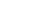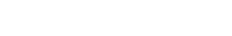# Chemistry Paper 1 - 2021 KCSE Prediction Questions and Answers Set 1

### Instructions to Candidates

1. Attempt all questions
2. Silent electronic calculators may be used.
3. All working must be clearly shown where necessary

1.
1. State Boyle’s law [1mk]
2. At 4000C, 850cm3 of a gas exert a pressure of 560mmHg. What volume of the same gas would exert a pressure of 640mmHg at the same temperature?  [3mks]
1. When burning magnesium is lowered into a gas jar containing nitrogen (I) oxide, it continues to burn forming a white solid
1. Name the white solid                                                                                                     1mk]
2. Write a chemical equation of the reaction that occurred                                               [1mk]
1. Carbon {IV} oxide is one of the gases used in fire extinguishers
1. State any other possible use of carbon {IV} oxide                                                       [1mk]
2. Name any two reagents that can be reacted together ti generate carbon {IV} oxide   [2mks]
1. Rusting is a process that causes massive destruction of iron structures
1. State one condition that accelerates rusting                                                                   [1mk]
2. State one advantage of rusting                                                                                        [1mk]
1. At 600C, 38 grams of lead{II} nitrate saturate 56cm3 of water. Determine the solubility of lead {II} nitrate at this temperature  [2mks]
2. Explain why molten sodium chloride conducts electricity, but solid sodium chloride does not  [2mks]
1. A polymer can be represented as1. Name and draw the structure of the monomer                                                                [2mks]
2. What type of polymerization occurs in the above case?                                                   [1mk]
3. Given that the molecular mass of the polymer is 25620, how many units of the monomer make the polymer  [2mks]
1. A reaction can be represented as;
C2H4[g] +HBr[g]  →C2H5Br[g]
Given the bond energies of C-H, C=C, C-C, C-Br, and H –Br as 20kJ/mol, 580Kj/mole, 446Kj/mole, 438KJ/mole and 396kJ/mole respectively. Determine the heat of formation of C2H5Br                                                                                                                                 [3mks]
2.
1. Define the term, dynamic equilibrium                                                                       [1mks]
2. A reaction at equilibrium can be represented as
2CrO2-4[aq] +2H+[aq]Cr2O7 2[-aq] + H2O{l}
Yellow                                        orange
State and explain the observation made when;
1. NaOH is added to the equilibrium mixture     [2mks]
2. HCl is added to the equilibrium mixture          [2mks]
1. During the electrolysis of dilute copper {II} chloride using carbon electrodes, a current of 1.5A was passed through the solution for 2 hours and 30 minutes
1. Write the ionic equation of the reaction that occurred at the cathode                       [1mk]
2. Given R.A.M of copper = 64 and 1F = 96500C, calculate the change in mass of the cathode          [3mks]
1.
1. Define the term half-life                                                                                          [1mk]
2. Name two particles likely to be emitted when a radioactive nuclide undergoes radioactivity
3. The half-life of a radioactive nuclide is 3 hours. Given that its initial mass is 288g, determine the remaining mass after 12 hours.           [2mks]
1. The reduction potentials of elements M and N are;Using the above reduction potentials, predict whether a reaction would occur between N+[aq]     and M[[s]   [3mks]
1. An hydrocarbon can be represented as: CH2
1. Name the hydrocarbon                                                                                            [1mk]
2. State two reagents that can be reacted together to generate the hydrocarbon           [2mks]
3. Identify the group of hydrocarbons into which C2H2 belongs to                               [1mk]
1.
1. Name two allotropes of sulphur                                                                                [2mks]
2. In an experiment to investigate a certain property of sulphur, Maina added few drops of conc HNOto sulphur in a test tube and warmed the mixture
1. State one observation made                                                                                      [1mk]
2. Write a chemical equation of the reaction that occurred                                         [1mk]
1. Chlorine is commonly used in the manufacture of Ca (OCl)2
1. State one use of the above compound of chlorine                                                         [1mk]
2. Write a chemical equation leading to the production of Ca (OCl)2                               [1mk]
1. A compound can be represented as
1. What name is given to the above class of compounds                                                 [1mk]
2. Name two reagents that can be reacted together to generate the above compound    [2mks]
3. State two conditions necessary for the reaction leading to formation of the above compound to occur   [2mks]
1. Using dots and crosses, show bonding in carbon{II} oxide                                                   [2mks]
2. When 20g of a compound containing carbon, hydrogen and oxygen was burnt in the air, 29.3g of carbon{IV} oxide and 11.7g of water were produced. Determine its empirical formulae.{C=12, H=1 , O=16}          [3mks]
1. Few drops of hydrochloric acid were added into a test tube containing lead {II} Nitrate solution
1. State one observation made                                                                                          [1mk]
2. Write an ionic equation of the reaction that occurred in the test tube                           [1mk]
1. In the industrial manufacture of Ammonia one of the raw materials is nitrogen gas
1. Name one other raw material                                                                                       [1mk]
2. Name two possible sources of the raw material you have named in {a} above           [2mks]
3. Name two substances that can be used as catalyst in this process                               [2mks]
4. State one use of ammonia                                                                                            [1mk]
1.  Gas X and Y can be collected as shown below1. Name the method used to collect gas Y                                                                       [1mk]
2. How do densities of gas X and gas Y compare?                                                       [1mk]
3. Give an example of a gas that can be collected using the same method as gas Y   [1mk]
1. Element W has two isotopes W – 36 and W-40 which occur in the ratio x:4. Given that R.A.M of W is 37.25, find the value of x    [2mks]
2. Describe an experiment that can be used to determine whether a given sample of a liquid is pure  [2mks]
1. A given mass of gas T diffuses through a porous plug in 48 seconds while a similar mass of gas R diffuse in 70 seconds. Given that the density of gas T is 0.6g/cm3, find the density of gas R [2mks]
2. The electron configuration of elements A, B, C, D and E are as given below
 Element Electron configuration A 2, 8, 1 B 2, 8 C 2, 7 D 2, 8, 6 E 2, 8, 3

1. Which element has the highest electrical conductivity                                                   [1mk]
2. Which letter represents the most reactive metal                                                           [1mk]
3. Which letter represents the most reactive non-metal                                                     [1mk]## MARKING SCHEME

1.
1. Bolyles law states that volume of a given mass of a gas inversely proportional to its pressure at constant temperature
2. P1V1=P2V2
560 x 850 = V2
640
V2=743.75 cm3
1.
1. Magnesium oxide
2. Mg[s] + N2O[S] →   MgO + N2[g]
1.
1. Manufacture of fizzy drink
Used as a refrigerant
[any one correct]
2. Marble chips [solid calcium carbonate and dilute hydrochloric acid              (Any correct 2)
2.
1. Salty condition
Acidic condition
2.  -Addition of minerals to the soil
-decomposition of iron waste
1.1. Molten sodium chloride has mobile ions while solid sodium chloride does not have mobile ions
2.
1.3.  n = 25620
48            48
N=533.75 units
[580 X1]+420X4 +1 →1 + [420 X5]+1
580+1680 +396 →446+2100+438
+ 2656 →-2984
+2656 -2984
=328kJmol-1
4.
1. Dynamic equilibrium is attained when the rate of the forward reaction is equal to that of the reverse reaction
2.
1. The intensity of the yellow colour in the equilibrium mixture increased . Additional of NaOH reduces the concentration ofH+ ions hence equilibrium shifts to the left
2. the intensity of the yellow colour in the equilibrium mixture decreases. Additional of HCL increased the concentration of H+ ions hence equilibrium shifts to the right
1.
1. Cu2+[aq] + 2e- →Cu[s]
2. Q= It
=1.5x150x60
=13,500C
2moles of electrons are depositing 1 mole of Cu metal
1 mole of electrons=96500C
2 moles of electrons =
193000C deposited 64g of copper
13500
4.476g
1.
1. Half life of a radioactive isotope is the time taken for a given or number of nuclides to decay to half its original mass or number
2. Alpha particle
Beta particles
3. the number of half life 12/3 =4
[1 /2 ]4x288=18g
1.The reaction will not take place because the e.m.f is negative
2.
1. Ethyne
2. Calcium carbide and water
3. Alkynes
3.
1. Rhombic sulphur
Monoclinic sulphur
2.
1. Red brown gas of fumes were observed
2. S[s] + 6HNO3[aq]→H2SO4[aq] +6NO2[g]+2H2O
4.
1. Acts as a bleaching agent
2. 2Ca[OH]2[aq]+Cl2[g] →CaCl2[aq] +Ca(OCl)2{aq} +2H[g]
1.
1. Ester
2. propanol and methanoic acid
3. Concentrated sulphuric {IV}acid catalyst
Warming
2.1.1.
1. White precipitate was formed
2. Pb2[q] + 2Cl [aq] →PbCl2[s]
2.
1. hydrogen
2. Electrolysis of brine
3. Cracking of larger alkanes
Finely divided iron
Plantinum catalyst
4. Manufacture of nitrogen fertilizer
Used as a refrigerant
Softening of water
3.
1. Upward delivery
2. gas x is denser than gas y
3. Hydrogen, ammonia,methane
22.x+4 x 36x x +40+4=37.25 x [x + 4]
36x + 160 = 37.25x +149
36x – 37,25x=149 – 160
-1.25x=+11
-1.25 =1.25
X=8.8
1. A liquid is boiled when constant boiling point is maintained the liquid is pure
2.1.
1. E
2. A
3. C

• ✔ To read offline at any time.
• ✔ To Print at your convenience
• ✔ Share Easily with Friends / Students